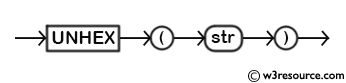# MySQL UNHEX() function

## UNHEX() function

MySQL UNHEX() function performs the opposite operation of HEX(). This function interprets each pair of hexadecimal digits (in the argument) as a number and converts it to a character.

This function is useful in -

• Hexadecimal to binary conversion: It allows you to convert a hexadecimal string representation into its binary form.
• Binary data manipulation: UNHEX() is often used to manipulate binary data stored as hexadecimal strings..

Syntax:

```UNHEX (str)
```

The return value is a binary string.

Argument:

Name Description
str A string which is to be converted to a decimal number.

Syntax Diagram:MySQL Version: 8.0

Example: MySQL UNHEX() function

```mysql> SELECT UNHEX('4d7953514c205475746f7269616c2c77337265736f757263');
+-----------------------------------------------------------+
| UNHEX('4d7953514c205475746f7269616c2c77337265736f757263') |
+-----------------------------------------------------------+
| MySQL Tutorial,w3resourc                                  |
+-----------------------------------------------------------+
1 row in set (0.01 sec)

mysql> SELECT UNHEX(HEX('w3resource'));
+--------------------------+
| UNHEX(HEX('w3resource')) |
+--------------------------+
| w3resource               |
+--------------------------+
1 row in set (0.00 sec)

mysql> SELECT HEX(UNHEX(1234));
+------------------+
| HEX(UNHEX(1234)) |
+------------------+
| 1234             |
+------------------+
1 row in set (0.00 sec)
```

In UNHEX() function the characters in the argument string must be legal hexadecimal digits i.e. '0'..'9', 'A'.. 'F', 'a'..'f'. If there are nonhexadecimal digits in argument part, the function will return NULL. See the following statement:

```mysql> SELECT UNHEX('www');
+--------------+
| UNHEX('www') |
+--------------+
| NULL         |
+--------------+
1 row in set (0.00 sec)```

All String Functions (Slides presentation)

Previous: UCASE
Next: UPPER

﻿